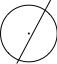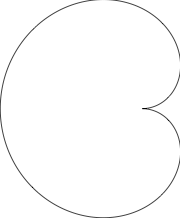# 有没有能用代码/命令行画图的工具?

MetaPost, Asymptote 都可以。

1 个赞

Inkscape，画好之后可以导出需要的代码

1 个赞
\documentclass{standalone}

\usepackage{tikz}

\begin{document}

\footnotesize
\begin{tikzpicture}
\def\a{.5}
\draw [help lines,step={\a}] ({-5.75*\a},{-5.75*\a}) grid ({5.75*\a},{5.75*\a});
\draw [thick,<->] ({-6*\a},0) -- ({6*\a},0) node [right] {x};
\draw [thick,<->] (0,{-6*\a}) -- (0,{6*\a}) node [above] {y};
\draw (0,0) circle ({4.5*\a});
\draw ({-5*\a},{-5*\a}) -- ({5*\a},{5*\a});
\foreach \x in {-5,-4,-3,-2,-1,1,2,3,4,5} {
\node at ({\x*\a},0) [below] {\x};
\node at (0,{\x*\a}) [left] {\x};}
\node at (0,0) [below right] {0};
\end{tikzpicture}

\end{document}


3 个赞
4 个赞

1 个赞

sagemath在美观程度上确实差一些，毕竟是基于python的matplotlib， 但是你可以看到代码非常简约，输入几乎就是题主说的半径，圆心以及直线上两点， 代码可读性也很高；另外sagemath结合python功能真心很强大。

1 个赞

# rotation参数是旋转角度
p += text("$y=x$", (2.1, 2.2),rotation=45.0, fontsize=16, color='red')


SVG 格式图片可以读写：

<svg version="1.1"
width="400" height="400"
xmlns="http://www.w3.org/2000/svg">

<rect width="100%" height="100%" fill="#fff" stroke="#000"/>

<circle cx="50%" cy="50%" r="100" fill="#fff" stroke="#f00"/>

<line x1="0" y1="0" x2="100%" y2="100%" stroke="#000"/>

<line x1="100%" y1="0" x2="0" y2="100%" stroke="#000"/>

</svg>1 个赞

3 个赞

asymptote 了解一下

Asymptote插件也可以在 GNU TeXmacs或者墨干编辑器中使用，我有一个比较

beginfig(3);
path a;
z = (12,30);
a = fullcircle scaled 2cm shifted z;
pickup pencircle scaled 1pt;
draw a;
draw (34,64)--(0,0);
pickup pencircle scaled 2pt;
drawdot z;
endfig;
end;beginfig(2);
u = 5cm;
draw for i=0 step 1 until 360: (sind(0.5i)*u*dir i).. endfor cycle;
endfig;
end;4 个赞

PGF 的基本命令和 MetaPost 是没啥差别的，能做的事是一样的。用 Mpost 好处是相比 PGF 不会严重拖慢编译速度，（有人做过比较，简单的图形 PGF 花时间是 Mpost 三倍，复杂些的可以到五倍）而且对比较新的 LuaTeX/ConTeXt 引擎 Mpost 是直接内建在引擎里还加了 lua 扩展支持，没有趋于淘汰的说法。

1 个赞